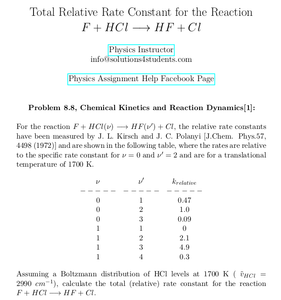Solved Problems in Physics, Physical Chemistry, and Computer Simulation Projects for College and Graduate Students.# Total Relative Rate Constant for the Reaction F + HCl → HF + Cl

Regular price
\$4.90
Sale price
\$4.90
Tax included.

Question:

For the reaction F + HCl(ν) → HF (ν') + Cl, the relative rate constants have been measured by J. L. Kirsch and J. C. Polanyi [J.Chem. Phys.57, 4498 (1972)] and are shown in the following table, where the rates are relative to the specific rate constant for ν = 0 and ν' = 2 and are for a translational temperature of 1700 K.Assuming a Boltzmann distribution of HCl levels at 1700 K ( ṽHCl = 2990 cm−1 ), calculate the total (relative) rate constant for the reaction F + HCl → HF + Cl.

This is the problem 8.8 of the book "Chemical Kinetics and Reaction Dynamics, written by Paul L. Houston".

By purchasing this product, you will get the step by step solution of the above problem in pdf format and the corresponding latex file where you can edit the solution. I am also available to help  you with any possible question you may have.